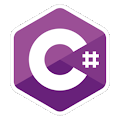Programming Course in C# ¡Free!

# Prime factors

Proposed exercise

Create a program that displays a number (entered by the user) as a product of its prime factors. For example, 60 = 2 · 2 · 3 · 5

(Hint: it can be easier if the solution is displayed as 60 = 2 · 2 · 3 · 5 · 1)

Output

Solution

```using System;
public class PrimeFactors
{

public static void Main()
{
int n;
int d = 2;

Console.Write("Enter the number: ");

while (n > 1)
{
while (n % d == 0 )
{
Console.Write(d);
Console.Write(" · ");
n = n / d;
}

d++;
}
Console.Write(1);
}
}
```